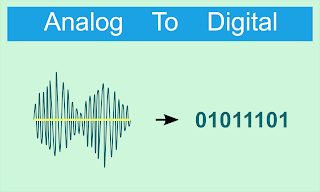How to Read ADC Values on Arduino UnoAnalog Digital Converter (ADC) is one of the processes for converting electrical voltages into digital values.The functions of this ADC vary from measuring voltage and displaying it to the LCD, as an audio recorder, as an analog signal translator, rotary encoder and so on.

The precision of an ADC conversion is indicated by the resolution. For example, an ADC with 8-bit resolution, can divide the 0-5Volt voltage into values 0-255. How this happens, consider the following calculations:

```11111111 = (1 × 2⁷) + (1 × 2⁶) + (1 × 2⁵) + (1 × 2⁴) + (1 × 2³) + (1 × 2²) + (1 × 2¹) + (1 × 2⁰) = 255
```

If given a voltage of 0-5volt, then the value of the voltage will be broken down into ADC values of 0-255. The higher the resolution of the ADC, the more accurate the readings obtained.

Currently, Texas Instruments has issued an ADC component with 32-bit resolution, meaning that if it is given a voltage of 0-5 volts, then the voltage value will be divided into ADC values from 0 - 4,294,967,295.

Very accurate isn't it? How about Arduino, what is the resolution of the ADC?

Arduino has an ADC with a 10-bit resolution, meaning that if it is given a voltage of 0-5V, the value that will be obtained from the ADC is 0-1023. In other words, 5V/1024 is 0.004Volt per sample.

How do we read the voltage and display it with digital data? In this tutorial, we will give an example of reading the ADC voltage using a potentiometer. Pay attention to the following picture:

Programs used:

```int adc = 0;

void setup()
{
Serial.begin(9600);
}

void loop()
{
}
```

After the above program is uploaded to Arduino, open the serial monitor and please rotate the potentiometer. Friends can be seen the value as follows:

### Converting ADC To Rated Voltage

Now, we will convert the ADC value into voltage. So that the voltage can be more precise, we use float. Here is the program:
```void setup() {
Serial.begin(9600);
}

void loop() {
float data = mapf(val, 0, 1023, 0, 5);

Serial.print(data);
Serial.println(" V");
delay(100);
}

float mapf(float value, float fromLow, float fromHigh, float toLow, float toHigh) {
float result;
result = (value - fromLow) * (toHigh - toLow) / (fromHigh - fromLow) + toLow;
return result;
}
```

Thank you for visiting the Chip Piko website. May be useful.